Next: Example of deconvolution with Up: HOW TO DIVIDE NOISY Previous: Dividing by zero smoothly

## Damped solution

Equation (12) is the solution to an optimization problem that arises in many applications. Now that we know the solution, let us formally define the problem. First, we will solve a simpler problem with real values: we will choose to minimize the quadratic function of x:(14)
The second term is called a damping factor" because it prevents x from going towhen.Set dQ/dx=0, which gives(15)
This yields the earlier answer.

With Fourier transforms, the signal X is a complex number at each frequency.So we generalize equation (14) to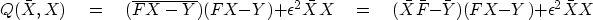(16)
To minimize Q we could use a real-values approach, where we express X=u+iv in terms of two real values u and v and then set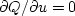and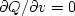.The approach we will take, however, is to use complex values, where we set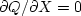and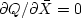.Let us examine:(17)
The derivativeis the complex conjugate of.So if either is zero, the other is too. Thus we do not need to specify bothand.I usually setequal to zero. Solving equation (17) for X gives equation (12).Next: Example of deconvolution with Up: HOW TO DIVIDE NOISY Previous: Dividing by zero smoothly
Stanford Exploration Project
10/21/1998